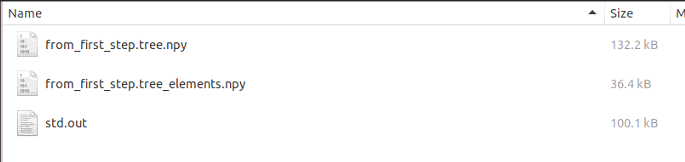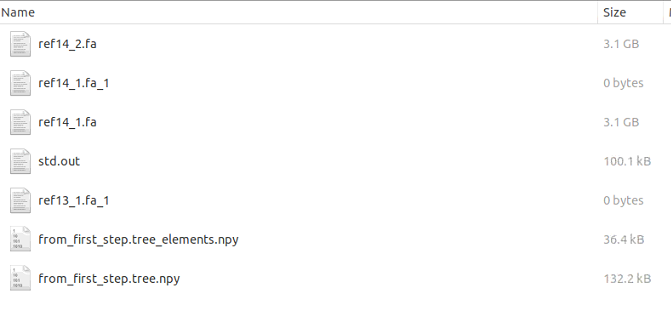macでインフォマティクス

# シングルセルDNAシーケンシングデータのシミュレータ SimSCSnTree

細胞の進化ツリーを生成し、その枝に沿って一塩基変異（SNVs）やコピー数異常（CNAs）を進化させる新しいシングルセルDNA配列シミュレータSimSCSnTreeについて報告する。このシミュレータで生成されたデータは、特にSNVやCNAが偏在するガンのシングルセルゲノム解析ツールのベンチマークに利用することができる。

SimSCSnTreeは現在BioCondaで公開されており、また、https://github.com/compbiofan/SimSCSnTree.git、詳細なドキュメントとともに自由にダウンロードできる。

インストール

• Python 3 or up.
• Python modules: numpy 1.18 or above, anytree.

Github

`#condamamba create -n simscsntree -yconda activate simscsntree#依存mamba install -c bioconda wgsim#本体mamba install -c bioconda simscsntree -ygit clone https://github.com/compbiofan/SimSCSnTree.gitcd SimSCSnTree/`

python main.par.overlapping.py

A single cell simulator generating low coverage data. The program automatically generates a phylogenetic tree with copy number variations on the branches. On each leave of the tree, it generates the reads whose error profile, such as uneven coverage, mimics the real single cell data.

Usage: python main.py -t [ref.fa] -n [number_leafs] -S [wgsim_dir]

-p (--processors)   Numbers of processors available.

-r (--directory)    Location of simulated data. The program will remove the whole directory if it already exists. Otherwise it will create one. (default: test)

-S (--wgsim-dir)    The directory of the binary of wgsim. It is in the same folder of this main.py. (need to specify)

-n (--cell-num)     Number of the cells on a level of interest. Always greater than -F treewidth. Treewidth controls the total number of clones whereas cell-num controls the total number of cells sequenced at a certain tree depth. (default: 8)

-B (--Beta)         The program uses the Beta-splitting model to generate the phylogenetic tree. Specify a value between [0, 1]. (default: 0.5)

-A (--Alpha)        The Alpha in Beta-splitting model. Specify a value between [0, 1]. The closer Alpha and Beta, the more balanced the tree. (default: 0.5).

-D (--Delta)        The rate of a node to disappear. Specify a value between [0, 1]. If all nodes have daughter nodes, take 0. (default: 0)

-G (--treedepth)    The mean of the tree depth distribution. The final tree depth will be sampled from a Gaussian with this mean and a fixed standard deviation. (default: 4 counting from the first cancer cell)

-K (--treedepthsigma)    The standard deviation of the tree depth distribution. To get exactly the tree depth defined by -F, use a very small standard deviation, e.g., 0.0001. (default: 0.5)

-F (--treewidth)    The mean of the tree width distribution. The final tree width will be sampled from a Gaussian with this mean and a fixed standard deviation. (default: 8)

-H (--treewidthsigma)    The standard deviation of the tree width distribution. To get exactly the tree width defined by -F, use a very small standard deviation, e.g., 0.0001. (default: 0.5)

-c (--cn-num)       The average number of copy number variations to be added on a branch. (default: 1)

-d (--del-rate)     The rate of deletion as compared to amplification. (default: 0.5)

-m (--min-cn-size)  Minimum copy number size. (default: 200,000bp)

-e (--exp-theta)    The parameter for the Exponential distribution for copy number size, beyond the minimum one. (default: 0.000001)

-a (--amp-p)        The parameter for the Genometric distribution for the number of copies amplified. (default: 0.5)

-t (--template-ref) The reference file to sequence the reads.

-o (--outfile)      The standard output file, will be saved in output folder, just give the file name. (default: std.out)

-f (--fa-prefix)    The prefix of the alleles and read names. (default: ref)

-x (--Lorenz-x)     The value on the x-axis of the point furthest from the diagonal on the Lorenz curve imitating the real coverage uneveness. (default: 0.5)

-y (--Lorenz-y)     The value on the y-axis of the Lorenz curve imitating the real coverage unevenness. x > y. The closer (x, y) to the diagonal, the better the coverage evenness. (default: 0.4)

-v (--coverage)     The average coverage of the sequence. (default: 0.02)

-l (--readlen)      Read length for each read sequenced. (default: 35bp)

-w (--window-size)  Within a window, the coverage is according to a Gaussian distribution. Neighboring windows' read coverage is according to a Metropolis Hasting process. (default: 200000bp)

-u (--acceptance-rate)  The probability to accept a proposal in Metropolis Hasting. (default: 0.5)

-k (--skip-first-step)  If the alleles for all nodes have been made, the step can be skipped. Make it 1 then. (default: 0)

-R (--snv-rate)     The rate of the snv. snv-rate * branch-length = # snvs. (default: 1)

-X (--multi-root)   The multiplier of the mean CNV on root. (default: 4)

-W (--whole-amp)    If there is whole chromosome amplification, 1 as yes. (default: 1)

-C (--whole-amp-rate)   Whole amplification rate: rate of an allele chosen to be amplified (default: 0.2)

-E (--whole-amp-num)    Whole amplification copy number addition, which occurs to one allele at a time. (default: 1)

-J (--amp-num-geo-par)  Whole amplification copy number distribution (geometric distribution parameter: the smaller, the more evenly distributed). (default: 1)

-Y (--leaf-index-range) For parallele job submission. >= min, < max leaf index will be processed. min.max. This counts leaf nodes from 0. (default: -1)

-L (--levels)    This is for both tree inference and sampling ancestral nodes. If the user is interested in sequencing nodes on multiple levels, user can specify the levels of interest by separating them by semicolon. The first tumor cell/clone under the trunk has level 1. If counting from the bottom (leaf) of the tree, use minus before the number. For example, -1 is the leaf level. The range of the level should be within [-depth, depth]. Users can specify desired levels according to -G to know which levels are available. If that is the case, use a very small -K to make sure the depth is not smaller than the biggest level you specify. (default: -1)

-U (--bulk-levels)    The levels of the bulk sequencing separated by semicolon. The definition of the levels is the same as in -L. The default for this option is NA, meaning no bulk sequencing.

-V (--cov-bulk)    The coverage of the bulk sequencing. The same for all levels. This parameter is needed when -U is identified. (default: 30)

-M (--single-cell-per-node)    If this is on, each node represents one cell and there is no clonality in the node. In this case tree_width will be the same as n (leaf num). 1 is on. (default: 0)

１、ゲノムをノードとし、CNAやSNVをエッジとするツリーを生成する。

（マニュアルより）このコマンドは、約8枚のleaf を持つツリー (--treewidth 8 --treewidthsigma 0.001) を、ツリーデプス4 (--treedepth 4) で、ブランチにCNVとSNVの両方をインプットしたシミュレーションを行う。SNV率は2（-R 2）、CNVサイズはp=5Mbp（-e 5000000）と最小サイズ2Mbp（-m 2000000）の指数分布に従うように設定される。すべての.npyファイルは、カレントディレクトリ下のdataフォルダ(-r data)に保存される。リファレンスゲノムはここではhg19を指定する。

`python main.par.overlapping.py -r data --treewidth 8 --treewidthsigma 0.001 --treedepth 4 --treedepthsigma 0.001 -t hg19.fa -m 2000000 -e 5000000 -R 2 -M 1`
• -r     Location of simulated data. The program will remove the whole directory if it already exists. Otherwise it will create one. (default: test)
• -t     The reference file to sequence the reads.
• -o     The standard output file, will be saved in output folder, just give the file name. (default: std.out)
•  -n　 Number of the cells on a level of interest. Always greater than -F treewidth. Treewidth controls the total number of clones whereas cell-num controls the total number of cells sequenced at a certain tree depth. (default: 8)
• -F (--treewidth)    The mean of the tree width distribution. The final tree width will be sampled from a Gaussian with this mean and a fixed standard deviation. (default: 8)
• -H (--treewidthsigma)    The standard deviation of the tree width distribution. To get exactly the tree width defined by -F, use a very small standard deviation, e.g., 0.0001. (default: 0.5)
• -G (--treedepth)    The mean of the tree depth distribution. The final tree depth will be sampled from a Gaussian with this mean and a fixed standard deviation. (default: 4 counting from the first cancer cell)
• -K (--treedepthsigma)    The standard deviation of the tree depth distribution. To get exactly the tree depth defined by -F, use a very small standard deviation, e.g., 0.0001. (default: 0.5)
• -m (--min-cn-size)  Minimum copy number size. (default: 200,000bp)
• -e (--exp-theta)    The parameter for the Exponential distribution for copy number size, beyond the minimum one. (default: 0.000001)
• -M (--single-cell-per-node)    If this is on, each node represents one cell and there is no clonality in the node. In this case tree_width will be the same as n (leaf num). 1 is on. (default: 0)
•  -R (--snv-rate)    The rate of the snv. snv-rate * branch-length = # snvs. (default: 1)

data/２、選択されたノード上のゲノムからリードをサンプリングする。

`python main.par.overlapping.py -k 1 -r data -S wgsim-master/ --Lorenz-y 0.28 --template-ref hg19.fa -M 1 -L -1 -Y 0.1 -v 0.001 -U -1 -V 5 -l 70`
• -k     If the alleles for all nodes have been made, the step can be skipped. Make it 1 then. (default: 0)SimSCSnTree: a simulator of single-cell DNA sequencing data Get access Arrow
Xian Fan Mallory, Luay Nakhleh
Bioinformatics, Published: 21 March 2022